# Introduction to PyMC: estimating a mean¶

By Ben Vincent, http://www.inferencelab.com.

A walkthrough of how to use PyMC that I used for my own learning. Maybe it will be useful to others.

This is a simple first test of how we can use PyMC to implement Baye's equation. We will apply it to the problem of estimating the mean of some data, where the variance is known. Although, it is more common to talk about the precision of the distribution ($\tau$) which is the inverse variance, $\tau = \frac{1}{\sigma^2}$.

We define a simple generative model describing how all the variables relate $P(\mu, \tau ,data)$ and specify this model for a simple example.$P(\mu|data,\tau) \sim \mathrm{Normal}(\mu,\tau=1)$

$P(\mu) \sim \mathrm{Uniform}(-10,10)$

We then want to use this model for:

• Step 1 - data generation, drawing samples from $P(data|\mu, \tau)$, and
• Step 2 - parameter estimation, drawing samples from $P(\mu|data, \tau)$.

These two steps form parameter recovery which is an important thing to be able to do to have some degree of belief that your model can do sensible inference when working with real data, where there are unknown (latent) variables.

The precision of the distribution is always known, so it always appears on the right of the $|$ symbol. It is easy to extend this model to simultaneously make inferences about the mean and the variability, but here we keep it simple.

In Part 1, we use PyMC in the 'normal' way, which involves creating a PyMC model for each step. In Part 2, we see how we can create a single PyMC function that can cleverly work with whatever observed data it is provided with. Thanks to Chris Fonnesbeck for the idea of how to do this.

In [ ]:


In :
# do some set up
%matplotlib inline
import matplotlib.pyplot as plt
import numpy as np
import pymc


## PART 1 - creating PyMC models for each step¶

### Step 1: Generating data by drawing samples from $P(data|\mu,\tau)$¶

First, define our known parameters.

In :
true_mu = 0.0
sigma = 1;
tau = 1 / sigma**2


Build the a set of PyMC objects which form the model which samples from $P(data|param)$

In :
def generate():
#prior
mu = pymc.Uniform("mu", lower=-10, upper=10,
value=true_mu, observed=True)
# likelihood function
data = pymc.Normal("data", mu=mu, tau=tau)
return locals()


Now construct a PyMC MCMC object. We are calling the pymc.MCMC method, and we pass it the function we just created to define the PyMC objects.

In :
pymc_generator = pymc.MCMC(generate())


We can use sample by calling this MCMC object's sample method. Here we only want 100 generated data points, and we discard the first 500.

In :
pymc_generator.sample(iter=500+100, burn=500)

 [-----------------100%-----------------] 600 of 600 complete in 0.0 sec

Extract the generated data from the MCMC object. Then use plot the samples using a PyMC method.

In :
pymc.Matplot.plot( pymc_generator.data )

Plotting data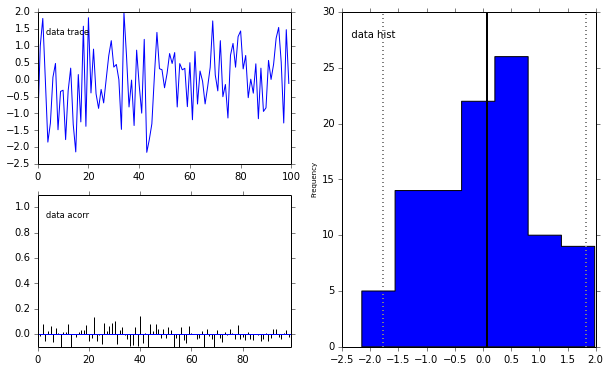### Step 2: Parameter recovery, sampling from $P(\mu|data, \tau)$¶

Now we have many data samples. How well can we make inferences about what the mean of the distribution is? We can use Baye's equation to infer what the probably parameter values were.

Now we will define a new PyMC model. Structurally, it is exactly the same as before. The only difference is that now the data are observed, and the parameter $\mu$ is not.

In :
# first, extract data out of the the object
# but we only want the first N samples
N=10
generated_data = pymc_generator.trace('data')[:N]
print(generated_data)

[-1.38129239  0.99114189  1.80995813  0.00485596 -1.84720602 -1.28637698
0.07006451  0.47904545 -1.48200903 -0.34934505]

In :
def inference():
#prior
mu = pymc.Uniform("mu", lower=-10, upper=10)
# likelihood function
data = pymc.Normal("data", mu=mu, tau=tau, value=generated_data, observed=True)
return locals()

# generate MCMC object
pymc_inference = pymc.MCMC(inference())

# do the inference
pymc_inference.sample(iter=500+100000, burn=500)

 [-----------------100%-----------------] 100500 of 100500 complete in 8.9 sec

Extract the samples of $\mu$ from the posterior $P(\mu|data,\tau)$ and plot them. We can see that the estimate is pretty good, in that the 95% credibility intervals overlap with the true parameter value that we defined above, $\mu=0$

In :
pymc.Matplot.plot( pymc_inference.mu )

Plotting mu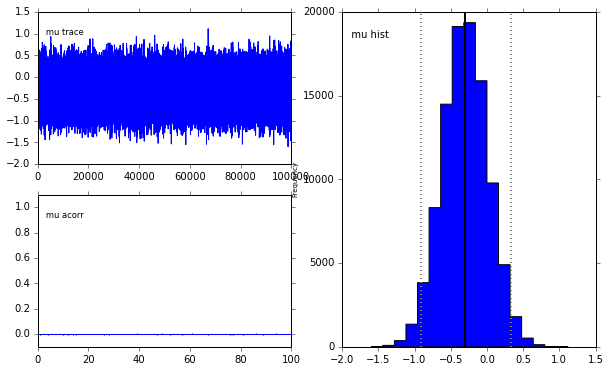## PART 2 - Creating a single PyMC model to do both these tasks¶

Above we had to explicitly define two different models to sample from two different conditional probability distributions from the same probabilistic model. For small models this is not really a big deal, but with larger models this could open the door for coding errors to be made.

So we can in fact just change the function defining the PyMC model to create a model which depends on what observed data we pass to it. The code below is an implementation of this, thaks to a suggestion made by Chris Fonnesbeck here (https://github.com/pymc-devs/pymc/issues/572).

In :
def generate_model(values):

#prior
mu = pymc.Uniform("mu", lower=-10, upper=10, value=values['mu'],
observed=(values['mu'] is not None))

# likelihood function
data = pymc.Normal("data", mu=mu, tau=tau, value=values['data'],
observed=(values['data'] is not None))

return locals()


### Step 1: generate data¶

In :
# specify what we know
observed = {'mu': true_mu,
'data': None}

# generate the model
pymc_generator = pymc.MCMC( generate_model(observed) )

In :
pymc_generator.sample(iter=500+100, burn=500)
pymc.Matplot.plot( pymc_generator.data )

 [-----------------100%-----------------] 600 of 600 complete in 0.0 secPlotting data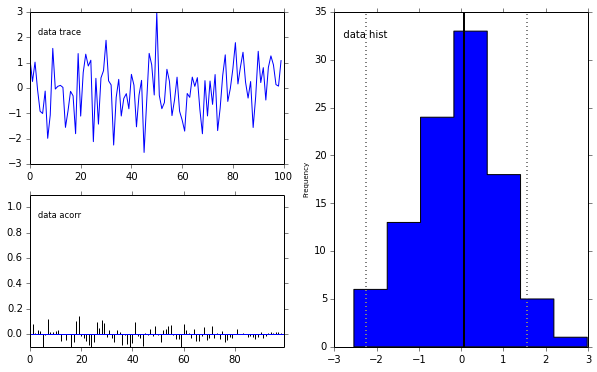### Step 2: Infer the mean¶

In :
# Extract first N data points from the step above
generated_data = pymc_generator.trace('data')[:N]
print(generated_data)

[ 1.14827482  0.26037412  1.02306693 -0.06213905 -0.91288403 -1.00143943
-0.11736827 -1.97964367 -1.0613446   1.5654022 ]


Now we will use the SAME generate_model() function, but simply pass it a different dictionary of observed values.

In :
# specify what we know, in the form of a dictionary
observed = {'mu': None,
'data': generated_data}

# generate the model
pymc_inference = pymc.MCMC( generate_model(observed) )

# do the inference
pymc_inference.sample(iter=500+100000, burn=500)

 [-----------------100%-----------------] 100500 of 100500 complete in 8.6 sec
In :
# plot the samples of mu
pymc.Matplot.plot( pymc_inference.mu )

Plotting mu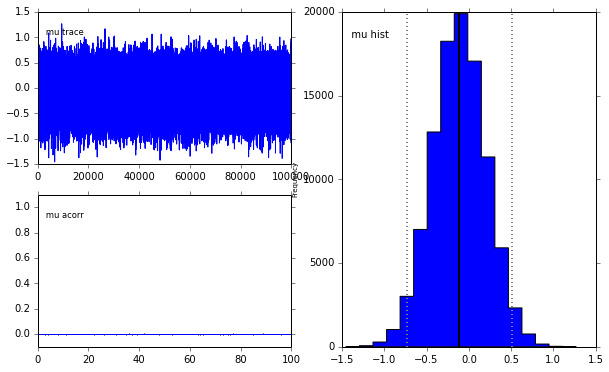Visualise the uncertainty in the inference by plotting Gaussian distributions centered on the inferred

In :
# Extract MCMC samples of mu
mu = pymc_inference.trace('mu')[:]

import scipy.stats as sp

plt.figure(1, figsize=(12,6))

x = np.linspace(-15,15,500)
sigma = (1.0/tau)**0.5

# plot the first 1000 samples
for i in mu[:1000]:
plt.plot(x, sp.norm.pdf(x,i,sigma), alpha=0.01, color='r')

# plot the data points on the bottom
plt.plot(generated_data, np.zeros(generated_data.shape)
,'+'
, c='k'
, markersize=20)

Out:
[<matplotlib.lines.Line2D at 0x10b8f8310>]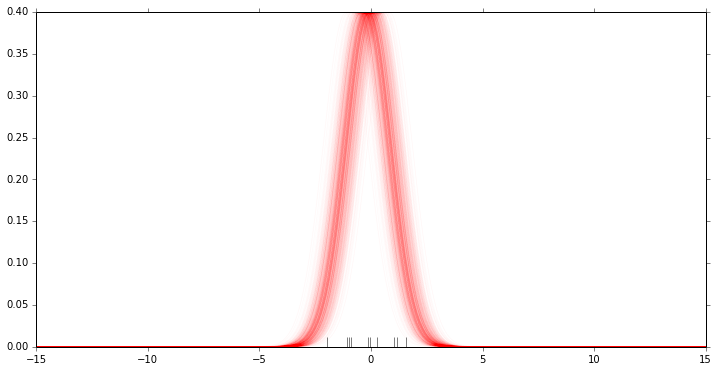In :